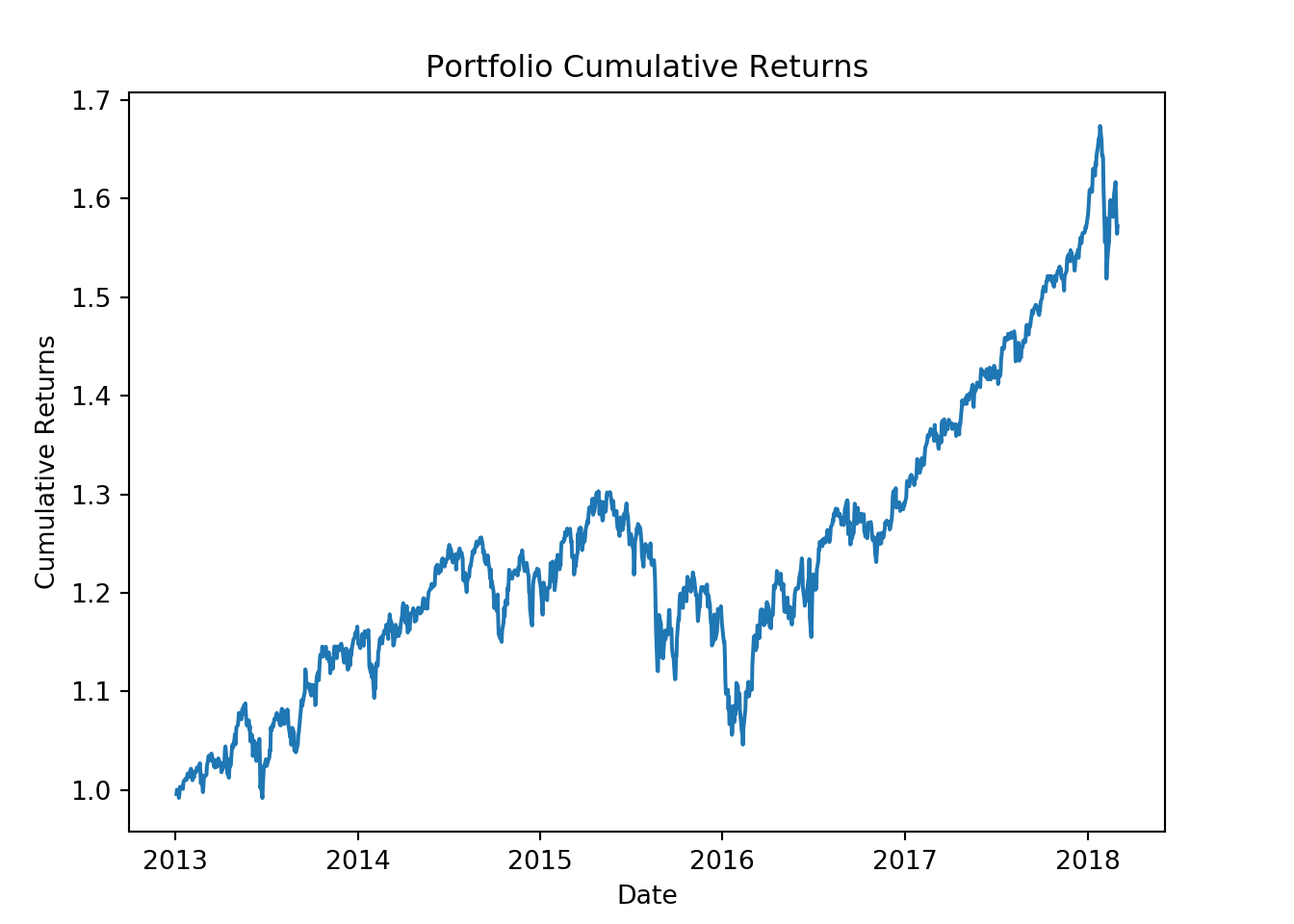## Calculating Cumulative portfolio returns in Python

In the last post we learned how to construct a portfolio in python. We also learned how to calculate the daily portfolio returns. In this post we will learn how to calculate portfolio cumulative returns.

First lets load the library.

``````import pandas as pd
import numpy as np
import matplotlib.pyplot as plt
import pandas_datareader as web``````

Then lets load the ticker symbols for our assets that we will include in our portfolio.

``````#  Assets to be included in the portfolio
tickers = ['BND', 'VB', 'VEA', 'VOO', 'VWO']``````

We will also create a vector for our asset weights.

``````# Asset weights
wts = [0.1,0.2,0.25,0.25,0.2]``````

Next lets download the price data from yahoo finance.

``````price_data = web.get_data_yahoo(tickers,
start = '2013-01-01',
end = '2018-03-01')
``````
``price_data = price_data['Adj Close']``

Next we will calculate the daily returns for our assets.

``ret_data = price_data.pct_change()[1:]``

We will then calculate the weighted average of our asset returns.

``````weighted_returns = (wts * ret_data)
``````## Symbols          BND        VB       VEA       VOO       VWO
## Date
## 2013-01-03 -0.000298 -0.000120 -0.002513 -0.000225 -0.001188
## 2013-01-04  0.000155  0.001516  0.001410  0.001049  0.000354
## 2013-01-07 -0.000072 -0.000597 -0.001262 -0.000671 -0.001723
## 2013-01-08  0.000096 -0.000383 -0.001339 -0.000748 -0.001648
## 2013-01-09 -0.000036  0.000864  0.001205  0.000750  0.001168``````

Finally the portfolio returns are the sum of the weighted returns.

``````port_ret = weighted_returns.sum(axis=1)
# axis =1 tells pandas we want to add
# the rows``````

To calculate cumulative returns we need to use the `cumprod()` function.

``cumulative_ret = (port_ret + 1).cumprod()``

Lastly we will plot our portfolios cumulative returns.

``````fig = plt.figure()